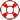Standard:

Description:

Standard:

# Math.4.NF.4b or 4.NF.B.4.B

Description:

## Understand a multiple of a/b as a multiple of 1/b, and use this understanding to multiply a fraction by a whole number. For example, use a visual fraction model to express 3 × (2/5) as 6 × (1/5), recognizing this product as 6/5. (In general, n × (a/b) = (n × a)/b.)4th Grade Math - Multiply by Fractions Lesson